### Mathematical Preliminaries: Mathematical Symbols

#### Learning Outcomes

• Identify the basic symbols of mathematics that will be used in the lecture
• Define sets
• Define functions

### Mathematical Symbols

The following are the mathematical symbols used throughout these online material.

• Curly brackets are used to designate sets. For example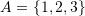is the set of the three elements 1, 2, and 3.
• We use the symbol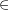to designate elements in sets. For example, if, then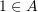.
• The symbol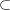means “subset of” and is used to indicate that the elements of one set are elements of another set. For example, ifand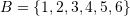, then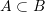.
• Sets can be defined using properties listed within the curly brackets. For example, we can define the set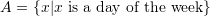. This means that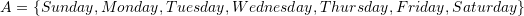.
•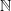is used to designate the set of natural numbers starting from 1. i.e.,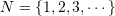.
•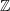is used to designate the set of integers. i.e.,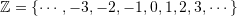.
•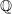is used to designate the set of rational numbers. i.e.,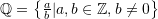.
•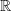is used to designate the set of real numbers.
• Box brackets are used to designate (closed) intervals in real numbers. For example,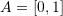is the set of real numbers between 0 and 1 and including both 0 and 1.
• Round brackets are used to designate (open) intervals in real numbers. for example,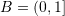is the set of real numbers between 0 and 1 and including 1.
• The symbol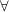means “for every” and is usually used to designate a property of all the elements of a set. For example, if we say that, we can describe the fact that all the elements in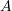are greater than zero by stating: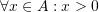.
• The symbol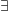means “exists” and is used to identify particular elements in sets. For example, ifwe can say that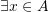such that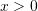, i.e., there exists an element in the setwhich is greater than 0.
• The symbol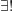means “exists and unique” and is used to identify a unique element in a set. For example, ifwe can say that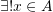such that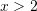, i.e., there exists one element and one element only in the setwhich is greater than 2.
• To define functions or maps between sets, we use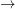. Ifis a set and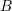is another set, then a function that maps elements ofinto elements ofis defined with the notation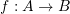.
• If, then, the arrow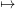is used to designate that a function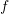maps the element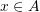to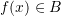as follows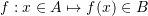.
• The symbol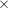is used in many contexts. One of them is the cross product which will be defined later. Another is for defining the set of ordered pairs. For example, ifandare two sets. Then, the set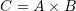is the set made of ordered pairs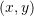withand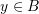. For example if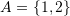and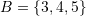, then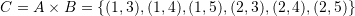. The set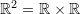is the set of ordered pairs of real numbers and geometrically designates a plane. Similarly,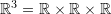is the set representing the three dimensional space.
•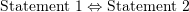is used to designate equivalence of statements 1 and 2(if and only if). For example: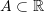is equivalent to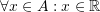. So, this can be written as: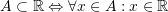.
•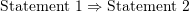is used to describe that statements 1 leads to statement 2. For example:leads to the statement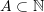. So, this can be written as: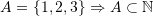.
• The Kronecker delta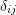is a special symbol which gives a value of either 0 or 1 depending on the subscriptsand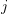. Usuallyandcan take values of 1, 2, or 3 and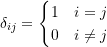• The alternator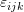is a special symbol which gives a value of either 0, 1, or -1 depending on the subscripts,, and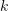. Usually,, andcan take values of 1, 2, or 3 and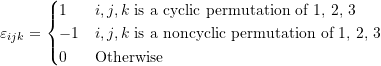• Unless stated explicitly, we do not differentiate between the column or row representation of vectors in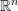. The curly brackets are also used for the row representation of vectors to be consistent with how Mathematica defines a vector. Therefore, the following are equivalent for the three dimensional vectorwith components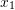,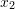, and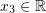: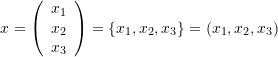• The reader should differentiate between vectors and sets depending on the context.

### Quiz 1 – Mathematical Symbols

1.Yibo Tang says:
2.Kenanao Sithole says:
3.Kamal Mohamed Noor says: